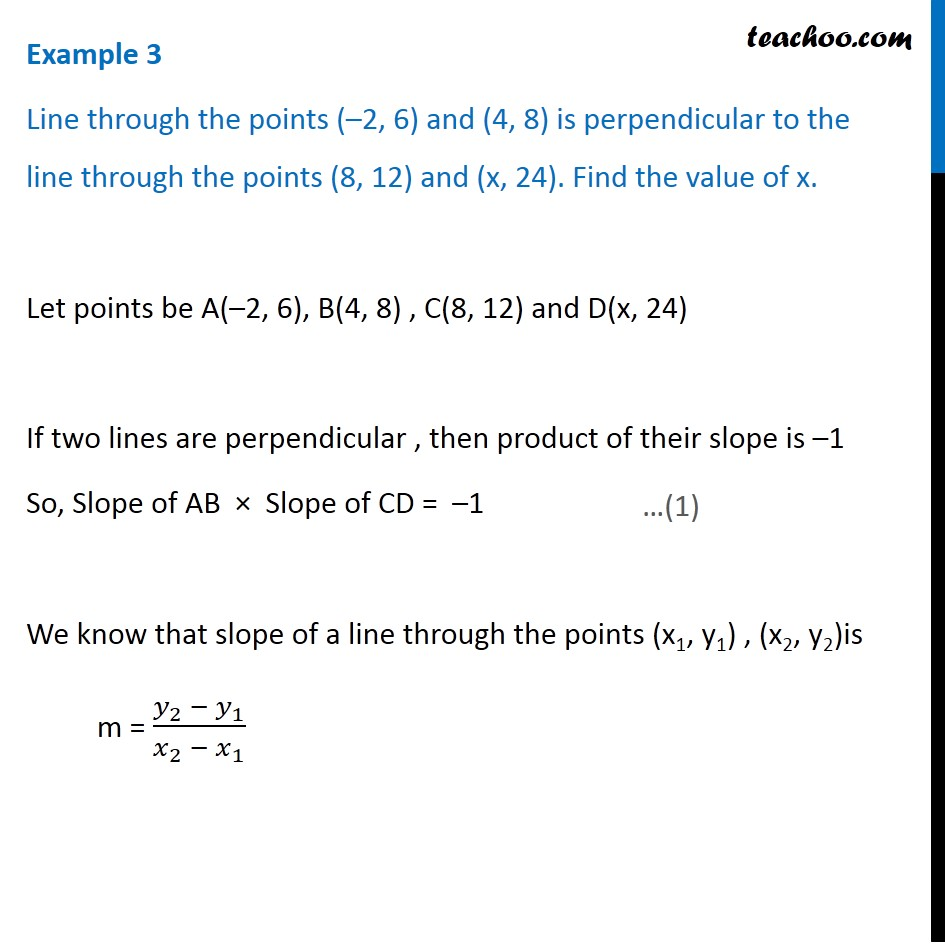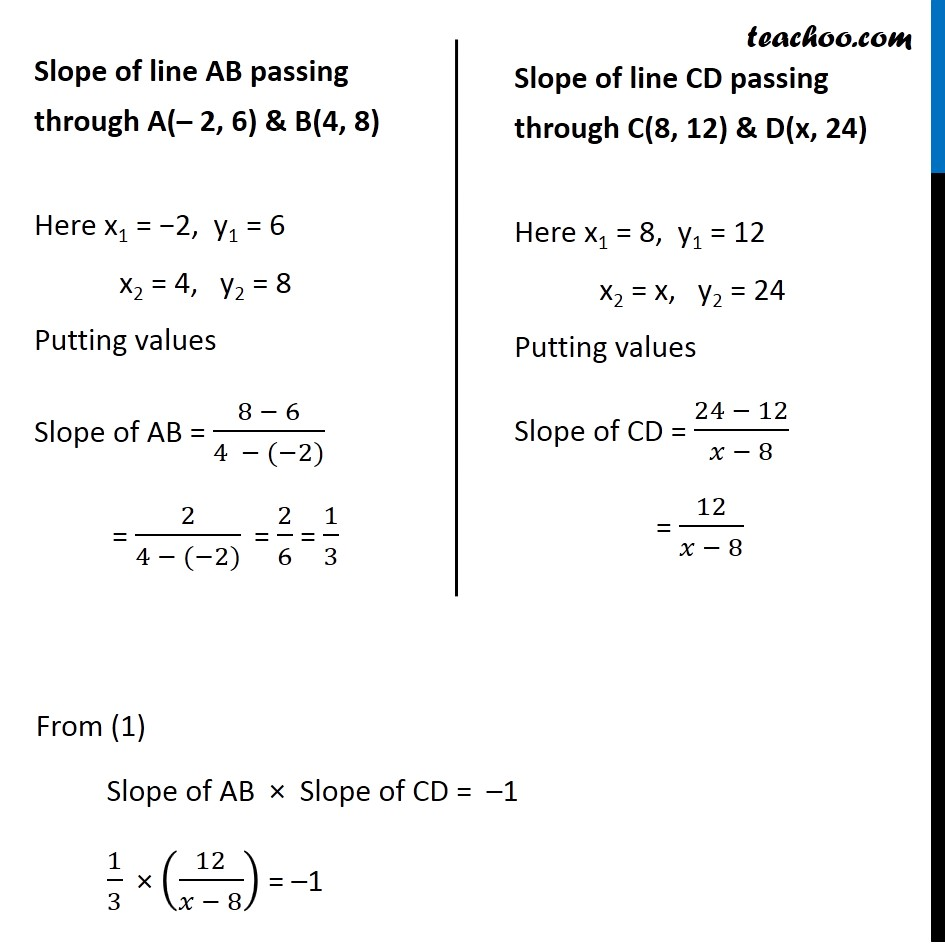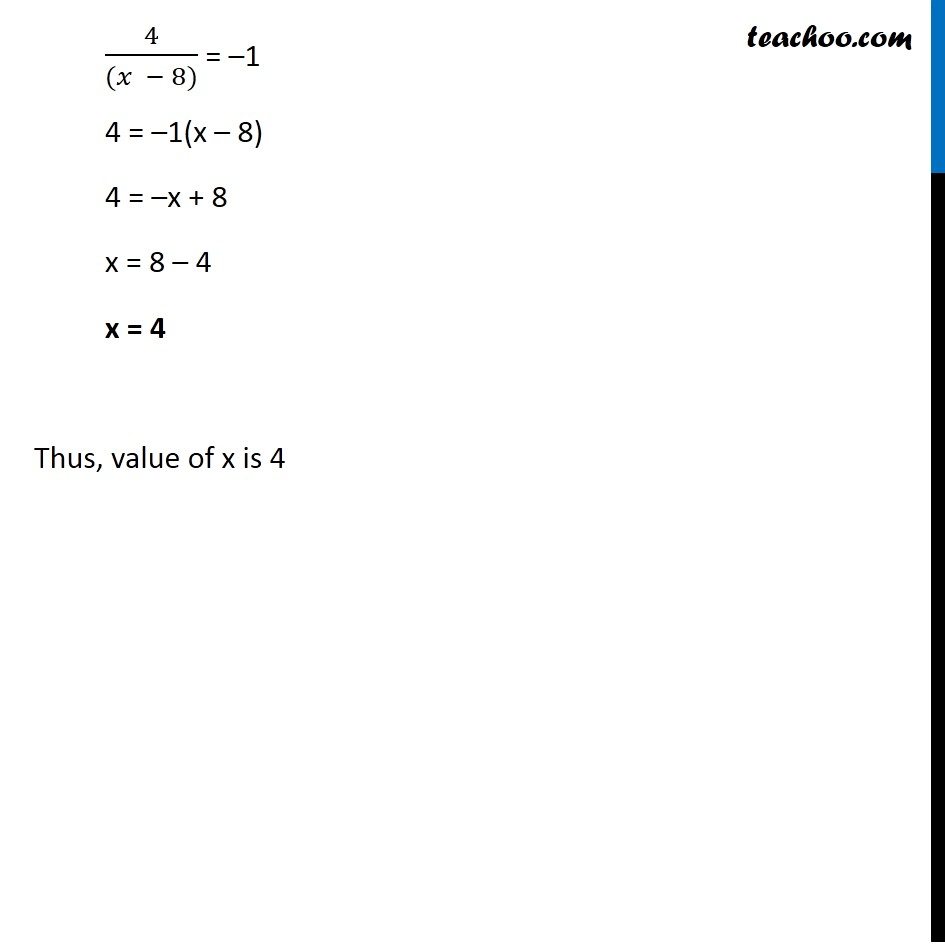Examples

Chapter 9 Class 11 Straight Lines
Serial order wiseLearn in your speed, with individual attention - Teachoo Maths 1-on-1 Class

### Transcript

Example 3 Line through the points (–2, 6) and (4, 8) is perpendicular to the line through the points (8, 12) and (x, 24). Find the value of x. Let points be A(–2, 6), B(4, 8) , C(8, 12) and D(x, 24) If two lines are perpendicular , then product of their slope is –1 So, Slope of AB × Slope of CD = –1 We know that slope of a line through the points (x1, y1) , (x2, y2)is m = (𝑦_2 − 𝑦_1)/(𝑥_2 − 𝑥_1 ) Slope of line AB passing through A(– 2, 6) & B(4, 8) Here x1 = −2, y1 = 6 x2 = 4, y2 = 8 Putting avalues Slope of AB = (8 − 6)/(4 − (−2)) = 2/(4 − (−2)) = 2/6 = 1/3 Slope of line CD passing through C(8, 12) & D(x, 24) Here x1 = 8, y1 = 12 x2 = x, y2 = 24 Putting values Slope of CD = (24 − 12)/(𝑥 − 8) = 12/(𝑥 − 8) From (1) Slope of AB × Slope of CD = –1 1/3 × (12/(𝑥 − 8)) = –1 4/((𝑥 − 8)) = –1 4 = –1(x – 8) 4 = –x + 8 x = 8 – 4 x = 4 Thus, value of x is 4